## 第三次作业

1.代码

#include<stdio.h>
main()
{int i,sum=0;
for(i=1;i<=100;i++) sum = sum+i;
printf("sum = %d",sum);
}

2.解题思路

（1)定义主函数

（2）定义两个变量，一个循环变量i，一个变量sum求和

（3）利用循环求1-100，如果大于100结束循环

（4）利用sum求1-100的和

（5）输出得数

3.流程图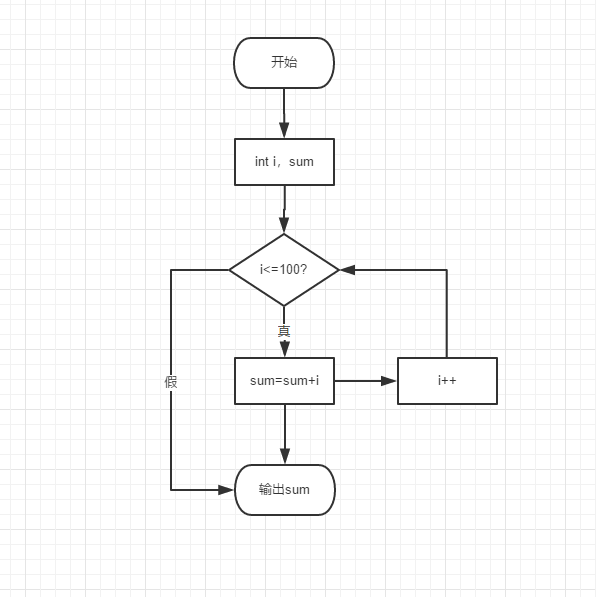4.运行结果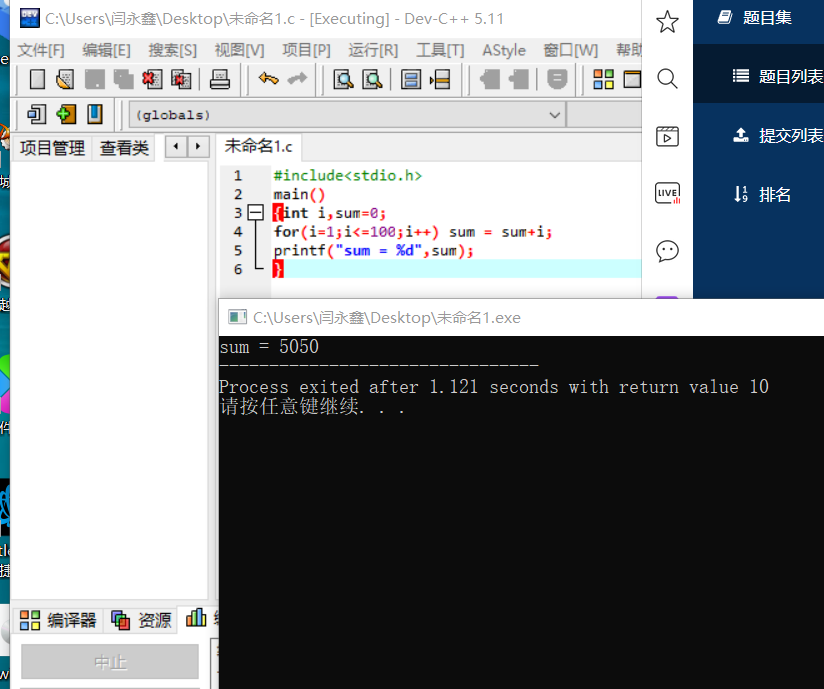5.调试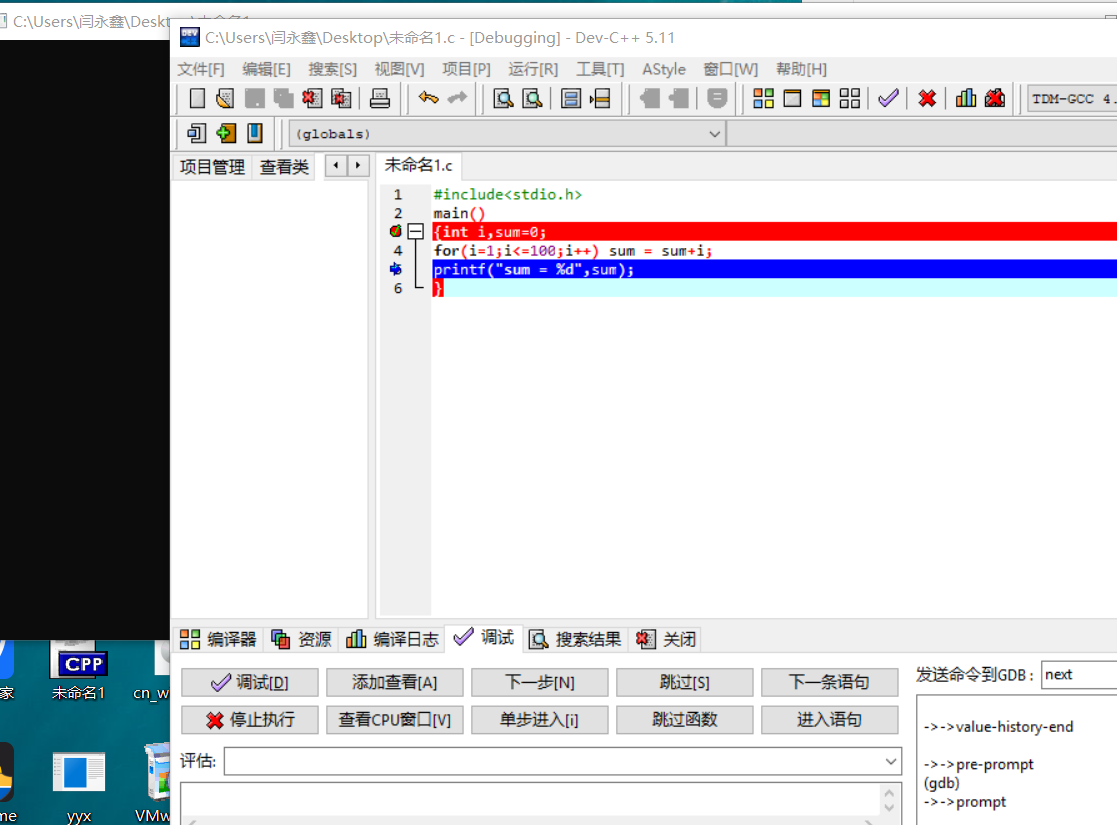6.总结

1.代码

#include<stdio.h>
int main()
{ int a,n;
while(a!=250){scanf("%d",&a);n++;}
printf("%d",n);
return 0;
}

2.解题思路

（1）定义一个函数

（2）定义一个循环变量a，和变量n（n用来计算寻找250的次数）

（3）写一个循环语句，随意输入一个数a，当a为250时结束循环，输出n。当n不为250时，继续循环，n+1

（4）输出n循环的次数

3.流程图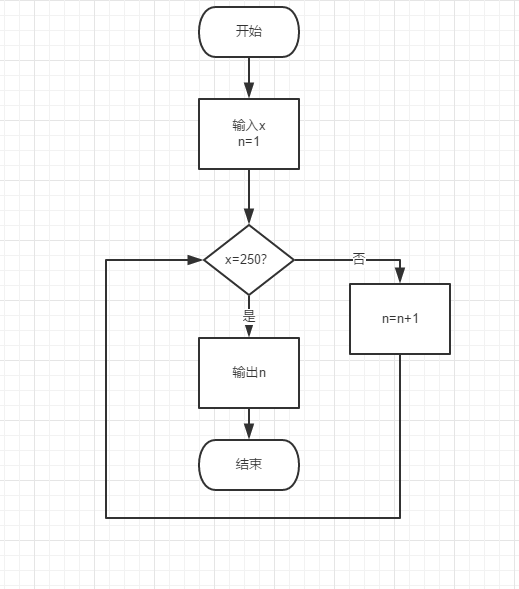4.运行结果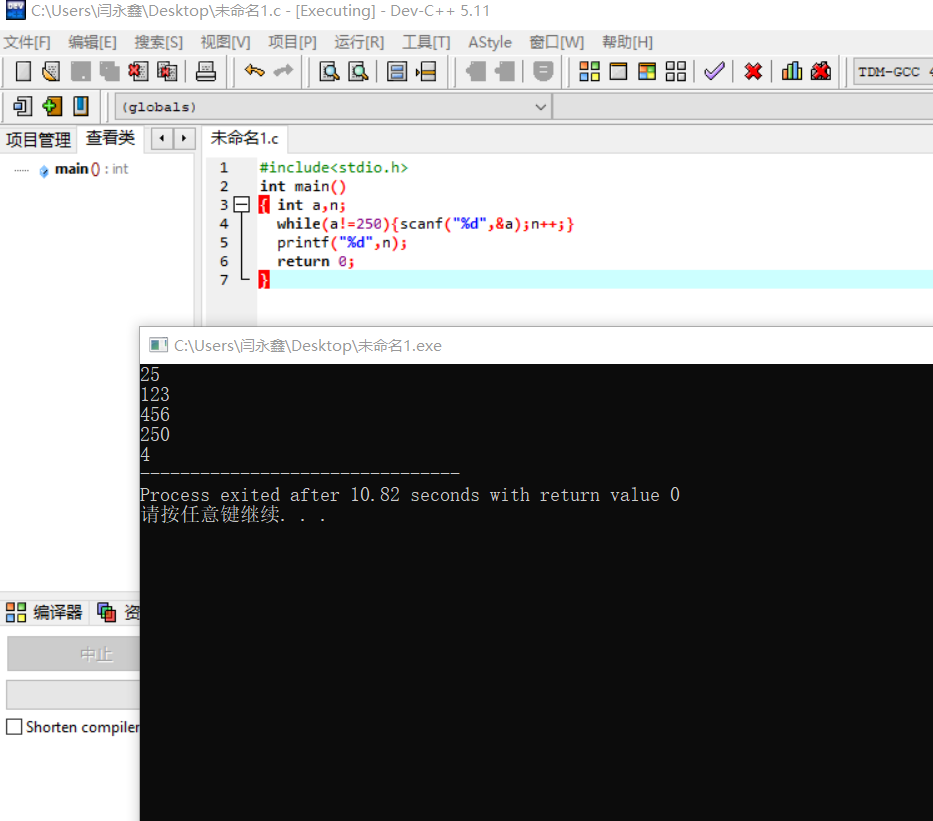5.调试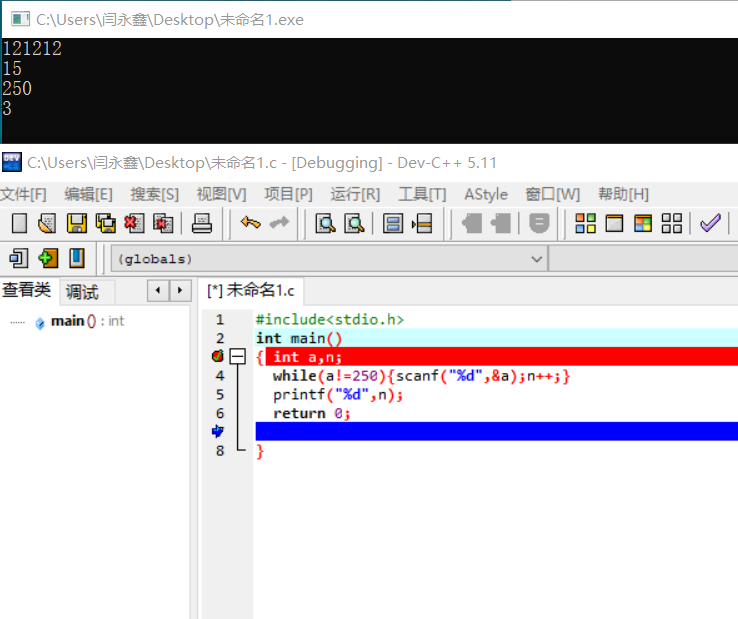6.总结

经过学习学会了新的循环语句while，不仅可以让循环更便捷，还可以让我们解决for解决不了的循环

1.代码

#include <stdio.h>

int sum(int m, int n);

int main()
{
int m, n;

scanf("%d %d", &m, &n);
printf("sum = %d\n", sum(m, n));

return 0;
}
int sum(int m,int n)
{
int sum=0;
for(; m<=n; m++){
sum=sum+m;
}
return sum;
}

2.结题思路

（1）定义一个子函数sum（sum为m到n的和）

（2）在主函数中调用m，n

（3）m<=n循环继续，，sum=sum+m（是求每次加上m得到的数），m=m+1（是使循环继续进行下去）

（4）当m>n时结束循环，并输出得数sum

3.流程图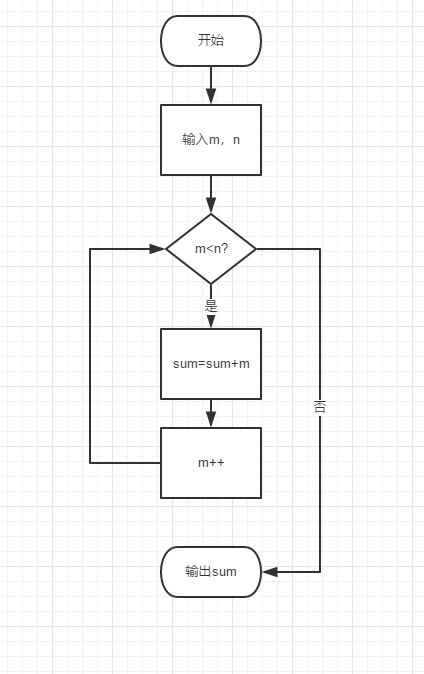4.运行结果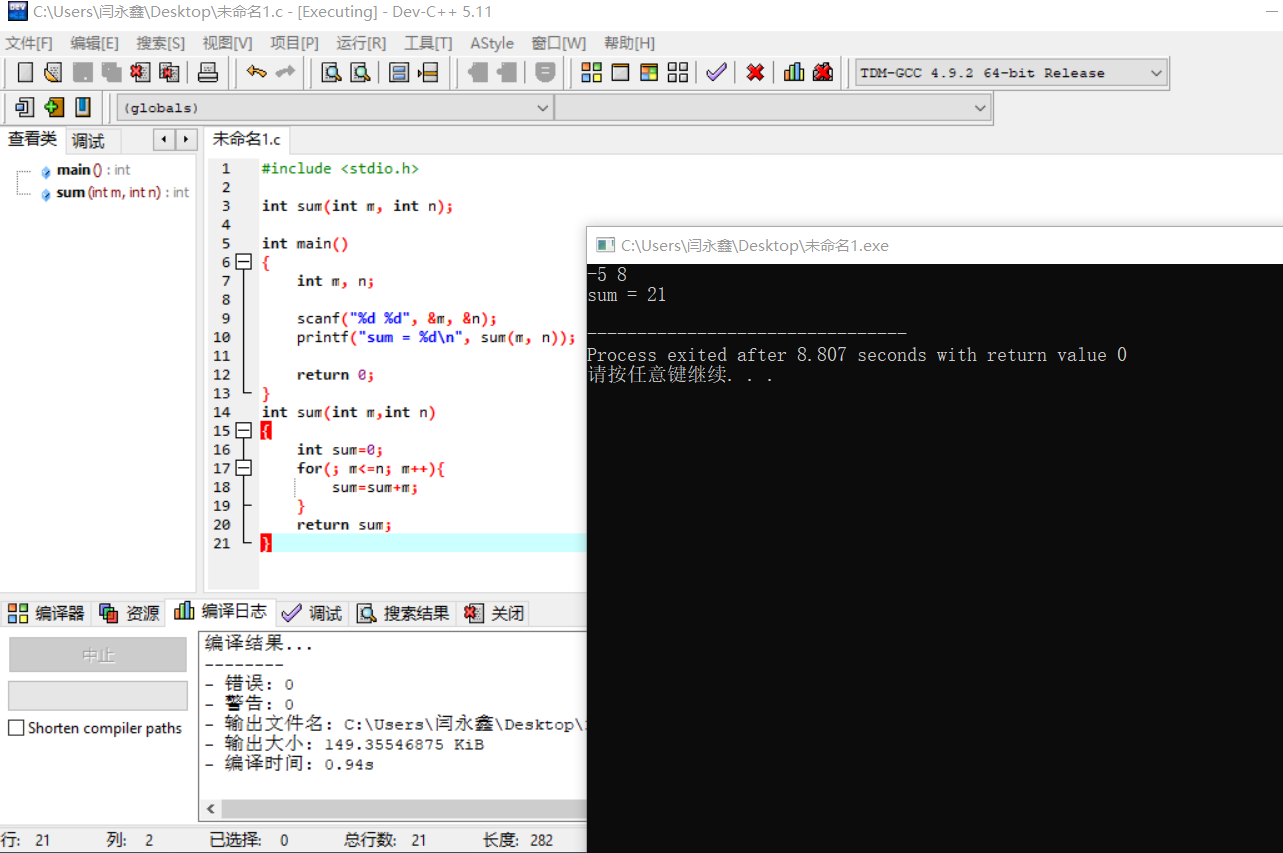5.调试6.总结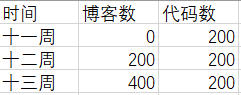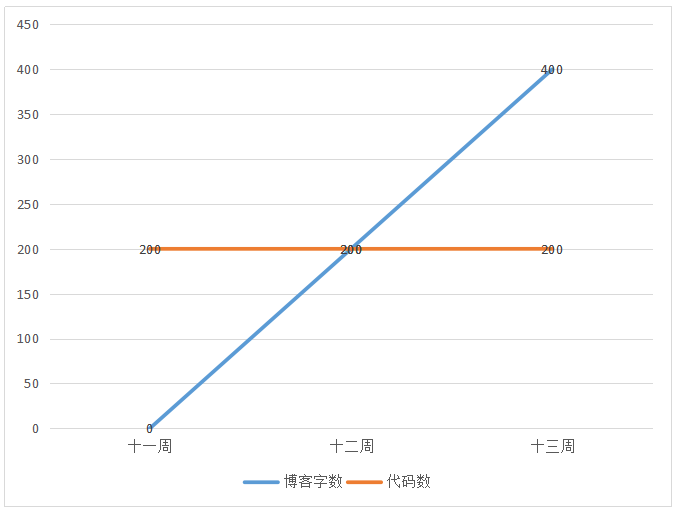https://www.cnblogs.com/159357www/p/10003543.html

https://www.cnblogs.com/zduangduang/p/10022227.html

https://www.cnblogs.com/chengxu230921/p/10017973.html

posted on 2018-12-01 00:06  闫永鑫  阅读(137)  评论(8编辑  收藏  举报